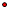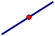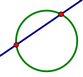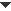﻿ Objects > Points

# PointsPoints are the fundamental building blocks of classical geometry, and geometric figures such as lines and circles are defined in terms of points. All of Sketchpad’s geometric constructions begin with points.

There are three kinds of points in Sketchpad.

 An independent point has no parents, and so does not depend on any other object. An independent point is free to move anywhere on the sketch plane.A point on a path is constructed on a path object such as a line or circle. A point on a path is free to move along its path, but cannot leave that path.A dependent point — such as a point of intersection — is constructed so that its position is completely determined by its parents. A dependent point cannot move by itself; it can move only if at least one of its parent objects also moves. Thus, a point at the intersection of two segments cannot move unless one or both of the intersecting segments are moved, and the reflected image of a point cannot move unless either the pre-image or the mirror moves.You can change the object properties, label properties, and display attributes of points.

Sketchpad provides a number of ways to create, manipulate, and use points.Construct a Point
 There are many different ways to construct points in a sketch. •Click the Point tool to construct a point.•Click the Straightedge tool or the Compass tool in empty space to construct a point that determines a straight object or circle. Many Custom tools also construct points.•Choose Construct | Point on Object to construct a point on each selected path object.•Choose Construct | Midpoint to construct a midpoint on each selected segment.•Choose Construct | Intersection to construct the intersection point of two selected objects. Each object must be a straight object, a circle, an arc, a point locus, or a function plot.•Choose Graph | Plot Value on Axis to plot a point on an axis or other path, at a position defined by a value or number.•Choose Graph | Plot Points to plot a point with coordinates defined by two values or numbers.You can also construct a point by using commands from the Transform menu to create a transformed image of an existing point.Drag, Animate, or Move a Point
 •Drag an independent point or a point on a path using the Translate Arrow tool, the Rotate Arrow tool, or thetool.•Animate an independent point or a point on a path using either the command or using an Animation button.•Move an independent point or a point on path toward a destination using a Movement button.When you drag or animate any other geometric object, it moves by dragging or animating the parents on which the object depends.Split or Merge a Point
 There may be times when you want to attach an independent point to another object. Similarly, there may be times when you want to separate a point from its parents to make it independent. The commands allow you to make such changes in the family tree of your sketch. •Choose Edit | Merge to merge an independent point with any other point or with a path, provided that the object to which it is being merged does not depend on the independent point.•Choose Edit | Split to separate any point on a path, midpoint, or intersection from its parents, making it into an independent point.•Choose Edit | Split Plotted Point from Coordinate System to split a plotted point from its defined coordinate values on its coordinate system.•Hold Shift and choose Edit | Split from Definition to split a transformed image point from its pre-image.See also: How to Use Split and Merge to Explore ConstructionsTransform a Point
 Transform a point by selecting it and choosing one of the following commands:Use Points to Define Transformations
 Use points in several ways to help specify how other objects will be transformed. •Choose Transform | Mark Center to designate a center point. The center point can be used for rotation or dilation.•Choose Transform | Mark Angle to designate an angle formed by three points. The angle can be used for translation or rotation.You can also designate an angle using two coterminal rays or segments. •Choose Transform | Mark Ratio to designate a point's value on a path or to designate a ratio defined by three collinear points. The ratio can be used for dilation.Measure a Point
 Several Measure menu commands apply to selected points or to combinations of points. •Choose Measure | Distance to measure the distance between two points.•Choose Measure | Angle to measure the angle formed by three points.•Choose Measure | Ratio to measure the ratio defined by three collinear points.•Choose Measure | Value of Point to measure the relative position of a point on a path.•Choose Measure | Coordinates to measure the coordinates of a point.•Choose Measure | Abscissa (x) to measure the x-coordinate of a point.•Choose Measure | Ordinate (y) to measure the y-coordinate of a point.